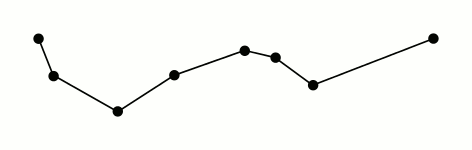# simplify(...)

### simplify(...)

Reduces the number of vertices in a line or polygon

Works with the following datatypes:

This function works with 2.1 endpoint(s). What does this mean? »

The simplify(...) function reduces the number of vertices in a line or polygon using the Ramer–Douglas–Peucker algorithm. This is useful for approximation or for reducing the total size of a geospatial dataset.simplify(...) takes two arguments:

• The geometry to be simplified
• The tolerance, in meters, used to simplify the segments in your geometry.

Be careful specifying a tolerance value that is too high, as you can actually simplify a geometry until it actually disappears. For a safer simplification method, try simplify_preserve_topology(...), which prevents the algorithm from oversimplification. For example, while simplify(...) will potentially collapse a circle until it disappears, simplify_preserve_topology(...) will at worst result in a triangle.

For example, to get a simplified version of the polygon for the City of Seattle from the Tiger LINE “Places” dataset: